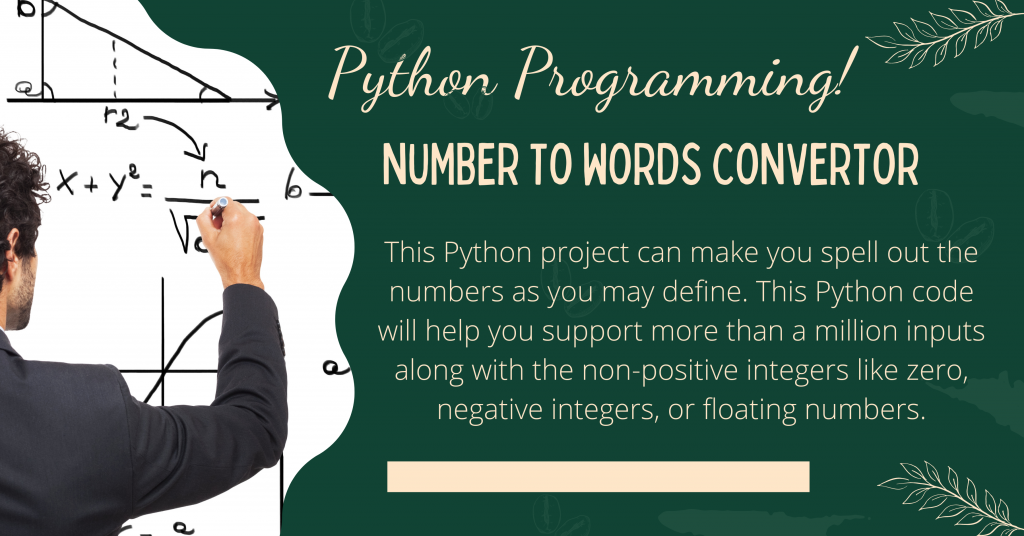# Python: Convert Number to WordsHello, Everyone! In this article, we’ll look at how to create a Python GUI project that converts integral values to words.

Let’s get started on the project straight away!

## Step 1: Importing Modules

We start off by importing all the necessary modules/libraries into our program.

We will be importing the `tkinter` module to create the GUI window. Along with this, we will be importing the `num2words` module in order to achieve the number to words functionality.

```import num2words as n2w
from tkinter import *
```

## Step 2: Create GUI Window

Now, we will be creating the basic Tkinter window using the basic functions and then add some basic widgets onto the screen. Some of them include Labels, Entry boxes, and buttons.

If you are unaware of Tkinter widgets, look at the tutorials mentioned below:

```root = Tk()
root.title("Numbers tdo Words")
root.geometry("650x400")
num = StringVar()
title = Label(root, text="Number to Words converter",fg="Blue", font=("Arial", 20, 'bold')).place(x=220, y=10)
formats_lable = Label(root, text="Formats supported :  ",fg="green", font=("Arial", 10, 'bold')).place(x=100, y=70)
pos_format_lable = Label(root, text="1. Positives :  ",fg="green", font=("Arial", 10, 'bold')).place(x=200, y=90)
neg_format_lable = Label(root, text="2. Negatives ",fg="green", font=("Arial", 10, 'bold')).place(x=200, y=110)
float_format_lable = Label(root, text="3. Zeros  ",fg="green", font=("Arial", 10, 'bold')).place(x=200, y=130)
zero_format_lable = Label(root, text="4. Floating points/decimals/fractions  ",fg="green", font=("Arial", 10, 'bold')).place(x=200, y=150)
num_entry_lable = Label(root, text="Enter a number :",fg="Blue", font=("Arial", 15, 'bold')).place(x=50, y=200)
num_entry = Entry(root,textvariable=num,width=30).place(x=220, y=200)
btn = Button(master=root, text="calculate",fg="green",
font=("Arial", 10, 'bold'),command=num_to_words).place(x=280,y=230)
display = Label(root, text="",fg="black", font=("Arial", 10, 'bold'))
display.place(x=10, y=300)
root.mainloop()
```

You might notice that in `Line 21` we have the `command` attribute of the button. The attribute is set to `num_to_words` but we haven’t declared the function yer.

## Step 3: The num_to_words function

In this function, we will be first reading the input given by the user and then convert the value read to words using the `num2words` function and finally changing the display value to the computed word form of the number.

```def num_to_words():
given_num = float(num.get())
num_in_word = n2w.num2words(given_num)
display.config(text=str(num_in_word).capitalize())
```

## Convert numbers to words using Python Tkinter

```import num2words as n2w
from tkinter import *

def num_to_words():
given_num = float(num.get())
num_in_word = n2w.num2words(given_num)
display.config(text=str(num_in_word).capitalize())

root = Tk()
root.title("Numbers tdo Words")
root.geometry("650x400")
num = StringVar()
title = Label(root, text="Number to Words converter",fg="Blue", font=("Arial", 20, 'bold')).place(x=220, y=10)
formats_lable = Label(root, text="Formats supported :  ",fg="green", font=("Arial", 10, 'bold')).place(x=100, y=70)
pos_format_lable = Label(root, text="1. Positives :  ",fg="green", font=("Arial", 10, 'bold')).place(x=200, y=90)
neg_format_lable = Label(root, text="2. Negatives ",fg="green", font=("Arial", 10, 'bold')).place(x=200, y=110)
float_format_lable = Label(root, text="3. Zeros  ",fg="green", font=("Arial", 10, 'bold')).place(x=200, y=130)
zero_format_lable = Label(root, text="4. Floating points/decimals/fractions  ",fg="green", font=("Arial", 10, 'bold')).place(x=200, y=150)
num_entry_lable = Label(root, text="Enter a number :",fg="Blue", font=("Arial", 15, 'bold')).place(x=50, y=200)
num_entry = Entry(root,textvariable=num,width=30).place(x=220, y=200)
btn = Button(master=root, text="calculate",fg="green",
font=("Arial", 10, 'bold'),command=num_to_words).place(x=280,y=230)
display = Label(root, text="",fg="black", font=("Arial", 10, 'bold'))
display.place(x=10, y=300)
root.mainloop()
```

## Some Sample OutputsSample Output 1 Number 2 WordsSample Output 2 Number 2 WordsSample Output 3 Number 2 Words

## Conclusion

I hope you understood the concept very well and enjoyed the outputs as well. Thank you for taking out time to read the tutorial.

Happy Learning! 😇